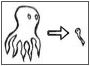ModelReduction.rudnyi.ru

 Home Model Reduction Applications Software
 MOR Home Linear Second Order Parametric Weakly Nonlinear Nonlinear

# Model Reduction of Second Order Systems

In many domains, the discretization of a partial differential equation leads to a system of ordinary differential equations of the second order. In principle, it can be always transformed to the first order and then model reduction methods for the first order system can be applied (flag -1 in mor4fem). However, the reduced system in this case is obtained as the first order ODEs and this is not always desirable.

In the special case of proportional damping, it is possible to ignore the damping matrix when the projection subspace is constructed but then to restore the reduced damping matrix from the reduced mass and stiffness matrices. The most interesting is that moment matching happens automatically for any values of alpha and beta, that is,in a way, we have parametric model reduction in respect to alpha and beta for free, without any extra efforts. This is the default behavior in mor4fem.

When damping is not proportional, one can derive second order Krylov subspaces in order to perform model reduction directly for the second order system. This happens to have many advantages as compared with the transformation to the first order system. mor4fem has a limited implementation of the SOAR algorithm (flag -2).

## Papers for Proportional Damping

E. B. Rudnyi, J. Lienemann, A. Greiner, and J. G. Korvink,
mor4ansys: Generating Compact Models Directly from ANSYS Models.
Technical Proceedings of the 2004 Nanotechnology Conference and Trade Show, Nanotech 2004, March 7-11, 2004, Boston, Massachusetts, USA, vol. 2, p. 279-282.

Model reduction of linear large-scale dynamic systems is already quite an established area. In a number of papers, the advantages of model reduction have been demonstrated. In the present paper, we describe a software tool to perform moment-matchingmodel reduction via the Arnoldi algorithm directly to ANSYS finite element models. We discuss the application of the tool to a structural mechanical problem with a second order linear differential equation (ODE). Its successful application to the first order case of electro-thermal modeling is demonstrated elsewhere.

J. S. Han, E. B. Rudnyi, J. G. Korvink.
Efficient optimization of transient dynamic problems in MEMS devices using model order reduction.
Journal of Micromechanics and Microengineering, 2005, v. 15, N 4, p. 822-832.
Paper at IOP.

One of the main obstacles to including transient dynamic effects into the performance functions of a structural optimization for microelectromechanical systems (MEMS) is the high computational cost of each time-dependent response simulation. This paper focuses on the application of model order reduction techniques to optimal design so as to reduce the transient analysis time for the optimization process. To do this, our open-source software mor4ansys performs model order reductions via the block Arnoldi algorithm directly to ANSYS finite element models. We adopt a micro accelerometer as an example to demonstrate the advantages of this approach. The harmonic and transient results of a reduced-order model of the accelerometer yield very good agreement with that from the original high-dimensional ANSYS model. The use of reduced-order models within the optimization iterations produces almost the same results as those without model order reduction, and speeds up the total computation by at least an order of magnitude.

R. Eid, B. Salimbahrami, B. Lohmann, E. B. Rudnyi, J. G. Korvink.
Parametric Order Reduction of Proportionally Damped Second-Order Systems.
Sensors and Materials, v. 19, N 3, p. 149-164, 2007.
Abstract, Preprint.

In this paper, the structure-preserving order reduction of proportionally damped and undamped second-order systems is presented. The discussion is based on the second -order Krylov subspace method, and it is shown that for systems with proportional damping, the damping matrix does not contribute to the projection matrices, and that the reduction can be carried out using the classical Krylov subspaces. As a result of direct projection, the reduced-order model is parameterized in terms of the damping coefficients.

## Papers for Second Order Krylov Subspaces

T. J. Su and R. R. Craig.
Model-Reduction and Control of Flexible Structures Using Krylov Vectors.
Journal of Guidance Control and Dynamics, vol. 14, pp. 260-267, 1991.
Paper at AIAA.

Z. Bai and Y. Su.
Dimension Reduction of Second-Order Dynamical Systems via a Second-Order Arnoldi method.
SIAM J. Sci. Comput., Vol.26, No.5, pp.1692-1709, 2005.
Paper at SIAM.

Behnam Salimbahrami and Boris Lohmann.
Order reduction of large scale second-order systems using Krylov subspace methods.
Linear Algebra and its Applications, v. 415, N 2-3, p. 385-405.
Paper at ScienceDirect.